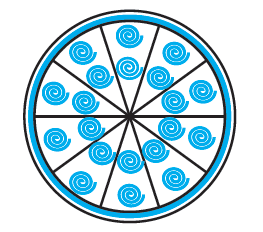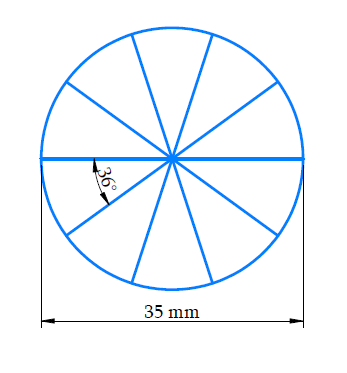# Ex.12.2 Q9 Areas Related to Circles Solution - NCERT Maths Class 10

Go back to  'Ex.12.2'

## Question

A brooch is made with silver wire in the form of a circle with diameter $$35\,\rm{mm.}$$ The wire is also used in making $$5$$ diameters which divide the circle into $$10$$ equal sectors as shown in the figure. Find:

(i) The total length of the silver wire required.

(ii) The area of each sector of the brooch.Video Solution
Areas Related To Circles
Ex 12.2 | Question 9

## Text Solution

What is known?

A brooch is made with silver wire in the form of a circle with diameter $$35 \,\rm{mm.}$$ The wire is also used in making $$5$$ diameters which divides the circle into $$10$$ equal sectors.

What is unknown?

(i) The total length of silver were required

(ii) Area of each sector of the brooch

Reasoning:

(i) Since the silver wire is used in making the $$5$$ diameters and perimeter of the circular brooch.

$$\therefore$$ Total length of silver were required

$$=$$ Circumference of circle $$+ \,5\, \times\,$$diameter

$$= \pi {{d}} + 5{{d}}$$ Where $${d}$$ is diameter of the brooch

$$={d}\,(\pi + 5)$$

(ii) To find area of each sector of the brooch, we first find the angle made by each sector at the centre of the circle.

Since the wire divides into $$10$$ equal sectors

$$\therefore$$  Angle of sector \begin{align} \theta =\frac{360^\circ }{10^\circ } \end{align}

Radius of the brooch \begin{align}= {\frac{d}{2}} \end{align}

$$\therefore$$ Area of each sector of brooch \begin{align}= \frac{\theta }{{{{360}^\circ }}} \times \pi {r^2} \end{align}

Steps:(i) Diameter of the brooch $${(d) = 35 \,\rm{mm}}$$

Total length of silver wire required $$=$$ Circumference of brooch $$+\, 5\,$$ diameter

\begin{align}&= \pi d + 5d\\& = (\pi + 5)35\,\\ &= \left( {\frac{{22 + 35}}{7}} \right) \times 35\\ &= 57 \times 5\\ &= 285\,\,\rm{{mm}}\end{align}

ii) Radius of brooch \begin{align}\left( r \right) = \frac{{35}}{2} = 17.5\,\rm{{mm}}\end{align}

Since the wire divides the brooch into $$10$$ equal sectors

$$\therefore$$ Angle of sector \begin{align} \theta = \frac{{{{360}^\circ }}}{{{{10}^\circ }}}\,\, = {36^\circ } \end{align}

$$\therefore$$ Area of each sector of the brooch

\begin{align}&= \frac{{{{36}^\circ }}}{{{{360}^\circ }}} \times \pi {r^2}\\& = \frac{1}{{10}} \times \frac{{22}}{7} \times 17.5 \times 17.5\\& = 2.2 \times 2.5 \times 17.5\\ &= 96.25\,\rm{{m}}{{{m}}^2}\end{align}

Learn from the best math teachers and top your exams

• Live one on one classroom and doubt clearing
• Practice worksheets in and after class for conceptual clarity
• Personalized curriculum to keep up with school